# Catch up on the latest AI articles# How To Prevent Overfitting In Adversarial Training

3 main points
✔️ Investigated the difference in error behavior between Normal and Adversarial Training
✔️ Found that overfitting is the dominant phenomenon in Adversarial Training
✔️ Showed that early stopping is effective for overfitting in Adversarial Training

written by Leslie RiceEric WongJ. Zico Kolter
(Submitted on 26 Feb 2020 (v1), last revised 4 Mar 2020 (this version, v2))

Subjects: Machine Learning (cs.LG); Machine Learning (stat.ML)code：## First of all

In recent years, it has become known that over-fitting can be suppressed by phenomena such as Double Descent in deep learning. However, in the case of Adversarial Training, which is a method for robustness against adversarial perturbations, we found that over-fitting is a dominant phenomenon, unlike in ordinary deep learning.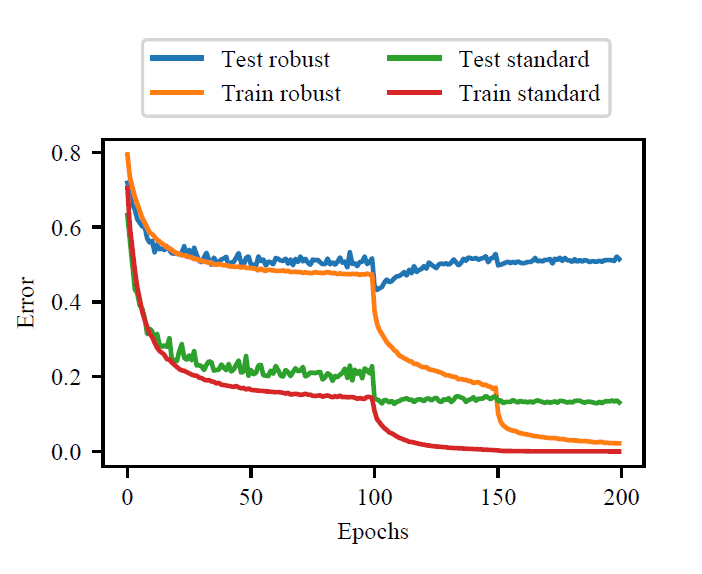In an example of training on CIFAR-10, as shown in the figure above, we can see that Double Descent occurs in normal training (Train standard, Test standard), but in Adversarial Training (Train robust, In Adversarial Training (Train robust, Test robust), the loss on the training data (Train robust) continues to decrease while the loss on the test data (Test robust) increases after a certain point. From this example, we can confirm that overfitting occurs when Adversarial Training is performed.

We find that early stopping is the most effective way to deal with such overfitting in Adversarial Training. We show that early stopping is the most effective method by comparing it with other regularization methods.

Adversarial Examples are input data that are designed to be misrecognized by the discriminative model. These adversarial examples are created by adding noise to the normal data. This noise is called adversarial perturbation, and various ways of making noise have been devised. The purpose of adding noise is to make the data misrecognized, i.e., to increase the error in classifying the data into the correct class. This can be formulated as follows.

$$max_{\delta \in \delta}l(f_{\theta}(x_{i} + \delta), y_{i})$$

where $l$ represents the loss function. As shown in this equation, we can create an adversarial perturbation by finding a perturbation $\delta$ that maximizes the loss when the model $f_{\theta}$ identifies the data $x_{i}$ with $y_{i}$. Solving this equation, we can create an adversarial sample, but it is not good if the sample is known to be adversarial by human eyes, so it is common to add constraints to the above optimization problem to add as small a perturbation as possible. There are various ways to measure the "smallness" of the perturbation here, but it is common to define the L1 norm, L2 norm, L∞ norm, etc. as the size of the perturbation and solve the above optimization problem while reducing the size of the perturbation.

Adversarial Training is a learning method to make a network robust against adversarial perturbations. The robustness is improved by training the network on adversarial samples, which are data generated by adversarial perturbations, in addition to normal training data. Formally, the parameter $\theta$ of the network is updated by the following rule. $$min_{\theta}\Sigma_{i}max_{\delta \in \delta}l(f_{\theta}(x_{i} + \delta), y_{i})$$ inner optimization problem, $max_{\delta \in \delta}l(f_{\theta}(x_{i } + \delta), y_{i})$ is an optimization problem to be solved when creating adversarial perturbations, and Adversarial Training This is called Adversarial Training.

## Experiment

There are several possible regularization methods to prevent overfitting in Adversarial Training. In this paper, besides early stopping, we investigate L2 regularization and data augmentation as candidates.

### Summary of results

Before going into the details, a summary of the results is provided.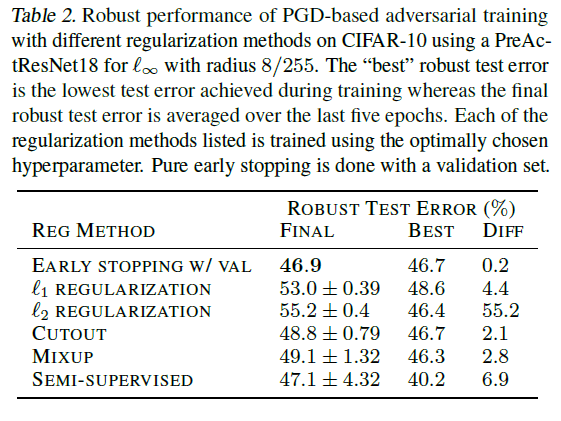This table shows that the early termination has the smallest robust test error.

### Premature termination

One of the problems in early stopping is what to stop the system based on. Therefore, we created learning curves for training data, validation data, and test data.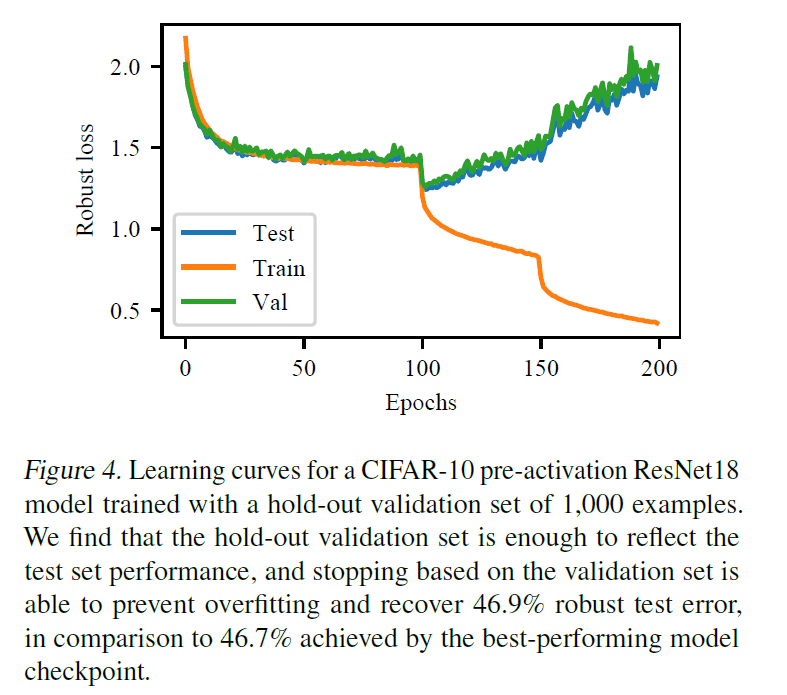According to this learning curve, we can see that the change of the loss for the validation data and the test data is almost the same, so we can reduce the loss for the test data if we terminate early when the loss for the validation data starts to increase.

### L2 regularization

The robust error on the test data (error on the test data with hostile samples) as the strength of the L2 regularization is varied is shown in the following figure.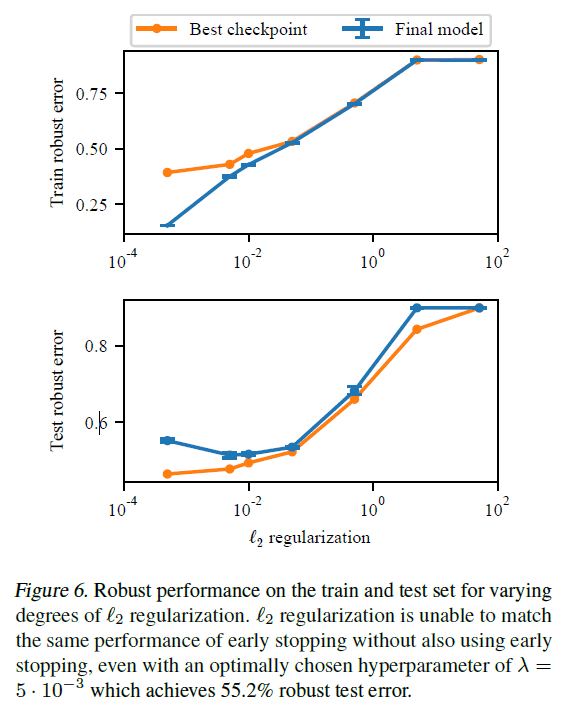With the optimal choice of hyperparameters, we achieve a robust error on the test data of 55.2%. However, this result does not extend to early termination.

### Data augmentation

We consider three methods of data augmentation: cutout, mixup, and semi-supervised learning.

Cutout

The change in the robust error as the length, a hyperparameter of Cutout, is varied is as follows.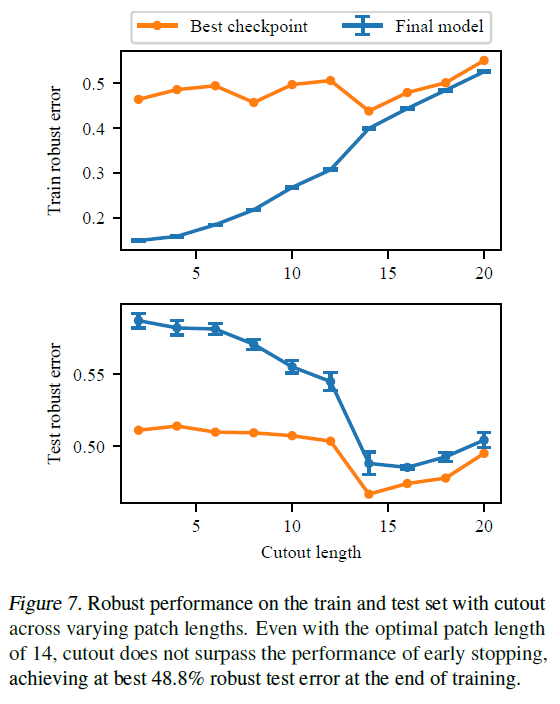For Cutout, the best score was 48.8% when Cutout length was set to 14, indicating that this was also not as good as an early termination.

Mixup

When the hyperparameter is changed in Mixup, the result is as follows.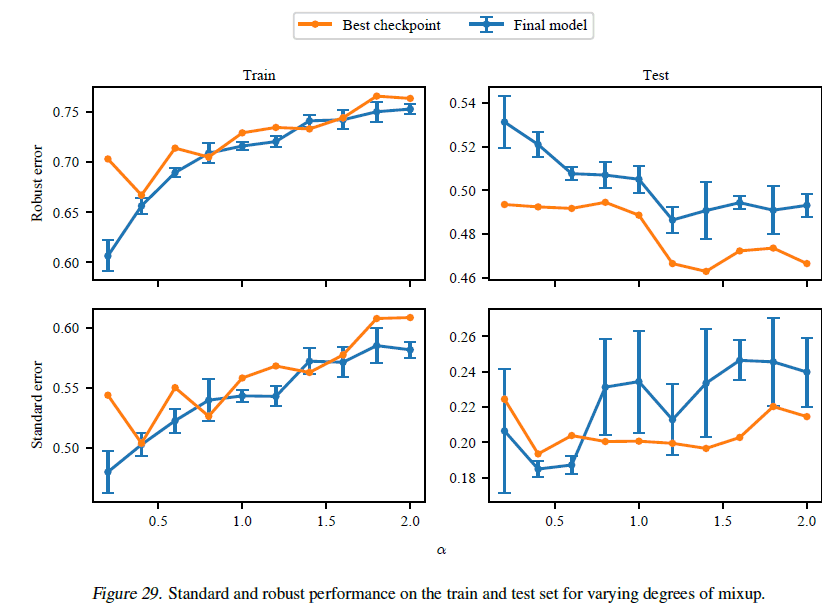As can be seen from the figure, Mixup does not terminate early even when the hyperparameters are changed. We can also see that there is a certain amount of variance in the results.

Semi-supervised learning

If we augment the training data with labeling in semi-supervised learning, we get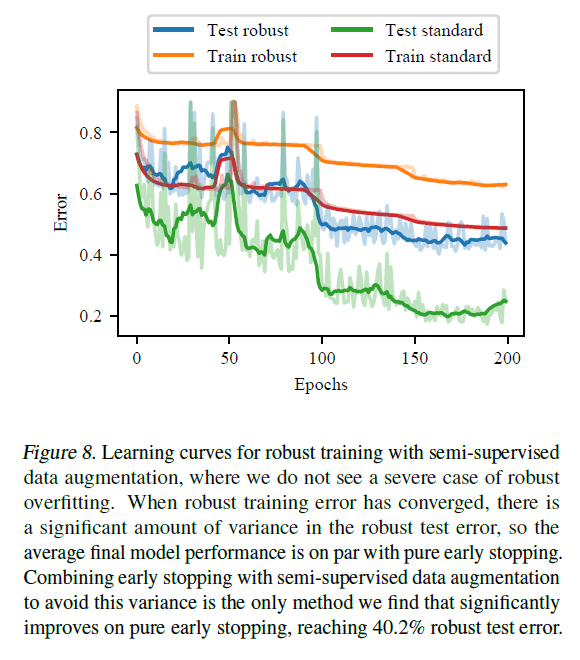Data augmentation with semi-supervised learning was the only method that performed as well as early termination on average, although the high variance of the results is a problem. The combination of data augmentation with semi-supervised learning and early termination results in a robust error of 40.2% on the test data, which is a significant improvement over pure early termination. Therefore, although data augmentation by semi-supervised learning is not as good as early termination when compared by itself, it is expected to have a significant effect when combined with early termination.

## Summary

We found that the error convergence properties of regular and adversarial training in deep learning are different. Most of the common regularization methods either over-regularize or allow over-fitting. Since overfitting is very likely to occur in Adversarial Training, it is important to check the learning curve carefully using validation data.

If you have any suggestions for improvement of the content of the article,